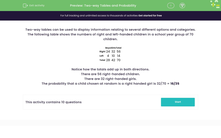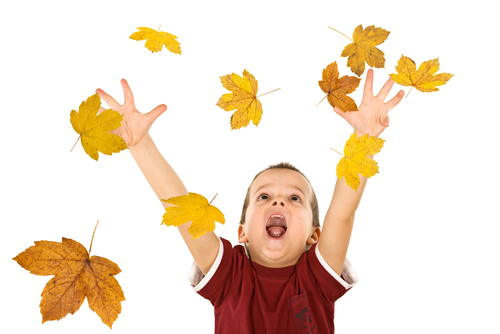# Use Two-way Tables to Find Probabilities

In this worksheet, students will read information from a two-way table to answer probability questions.Key stage:  KS 3

Curriculum topic:   Probability

Curriculum subtopic:   Calculate Theoretical Probability

Popular topics:   Probability worksheets

Difficulty level:#### Worksheet Overview

Two-way tables can be used to display information relating to several different options and categories.

The following table shows the number of right and left-handed children in a school year group of 70 children.

Boys Girls Total
Right 24 32 56
Left 4 10 14
Total 28 42 70

Notice how the totals add up in both directions.

There are 56 right-handed children.

There are 32 right-handed girls.

The probability that a child chosen at random is a right-handed girl is 32/70 = 16/35

Always remember to check that you have put the fraction into its simplest form.

Does that all make sense?  Then, let's get started.### What is EdPlace?

We're your National Curriculum aligned online education content provider helping each child succeed in English, maths and science from year 1 to GCSE. With an EdPlace account you’ll be able to track and measure progress, helping each child achieve their best. We build confidence and attainment by personalising each child’s learning at a level that suits them.

Get started